Next: 13. Simplification Up: 2. User's Guide Previous: 11. Theory Ensembles

Subsections

# 12. The Proof System

In purpose of this chapter is to explain:

• The IMPS proof system.
• A description of how you prove theorems in IMPS, in interactive mode and in script mode.
• A description of the proof-script language.

# 12.1 Proofs

A proof of a statement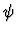is conclusive mathematical evidence of whyis true. A proof is usually thought of as a sequence of formulas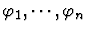such that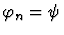and every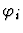is either:

1.
An axiom, that is, a formula which is assumed true to begin with;
2.
A theorem, that is, a formula whose truth has already been established by some other proof; or
3.
A consequence of one or more previous formulas in the sequence. This means thatis related to these formulas by a relation called an inference.
The criterion for determining what constitutes an acceptable inference depends on the purpose of the proof. If the proof is for mechanical verification only (whether by a digital computer or a human being acting like one), then the criteria of acceptability can be specified unambiguously as a set of rules for determining which sequences of symbols constitute proofs. This set of rules is called a proof system. For a human audience, on the other hand, it is usually a good idea to stress the essential or innovative steps in a proof and avoid routine computations. Moreover, for an expository article, a textbook, or a classroom lecture, it is acceptable, even desirable, that inference steps appeal to intuition.

# 12.2 The IMPS Proof System

In the traditional presentation of proofs in textbooks and journals, the deductive process appears as an inexorable progression from known facts to unknown ones. In contrast, the deductive process in IMPS begins with a conjecture and usually proceeds with a large amount of trial and error. This view of the deductive process leads naturally to the idea of a proof as a graph.

Proofs in IMPS are represented by a data structure called a deduction graph, which is a directed graph with two kinds of nodes: inference nodes and sequent nodes. A sequent node consists of a single formula called the assertion together with a context. The context is logically a set of assumptions, although the implementation caches various kinds of derived information with a context. An inference node i consists of a unique conclusion node c, a (possibly empty) set of hypothesis nodes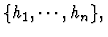and the mathematical justification for asserting the relationship between c and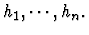This justification is called an inference rule.

As an interactive process, a proof consists of a series of reductions of unproven sequent nodes of the graph (which we think of as goals) to new subgoals. Each reduction is created by a procedure called a primitive inference procedure, which takes a sequent node and possibly other arguments, returns an inference node inf and, as a side-effect, changes the deduction graph. These changes include:

• The addition of inf to the set of inference nodes of the deduction graph.
• The addition of the hypotheses of inf to the set of sequent nodes.

There are about 25 primitive inference procedures. Primitive inference procedures are the only means by which inference nodes can be added to a deduction graph. As a user of IMPS, however, you will never apply primitive inference procedures directly; you will only apply them indirectly by applying proof commands--convenient procedures which add sequent nodes and inference nodes to a deduction graph by calling primitive inferences. Proof commands are documented in Chapter 18.

# 12.3 Theorem Proving

There are two modes of proving theorems in IMPS: interactive mode and script mode. Interactive mode is used for proof discovery and interactive experimentation with new proof techniques. Script mode is used primarily for proving theorems whose proofs are similar to previously constructed proofs or for checking a large number of proofs.

To begin an interactive proof, select the Start dg option in the menu or use the Emacs command M-x imps-start-deduction. IMPS will then prompt you in the minibuffer with the text Formula or reference number: which you can supply by clicking the right mouse button on the formula you want to prove (provided it is enclosed between double quotes) or by typing the reference number of the formula (provided it has already been built). All the proof commands and node-movement commands given during the course of a single proof are recorded as a component of the deduction graph. These commands can be inserted as text into a buffer (provided the major mode of the buffer is scheme mode) by selecting the option Insert proof script or by entering C-c i. The text that is inserted in the buffer is a script of proof commands which can be edited and used in other proofs. To run a command script, select the option Execute region or enter C-c r. This will run the commands in the current region. Note that an interactive proof can be combined with one or more proof segments run in script mode. This is especially useful for redoing similar arguments.

## 12.3.1 Checking Proofs in Batch

To check a large number of proofs, (for example, to check an addition to the theory library) proof scripts can be run in batch by using the shell command
   $IMPS/../bin/run_test testfile logfile  where testfile is a text file which may contain any def-form. Typically, it will consist of a series of include-files and load-section forms. The file logfile will contain the output of the process. For example, entering on a machine called veraguas the shell command $IMPS/../bin/run_test testy loggy

where testy contains
   (load-section abstract-calculus)

will produce a logfile loggy which will look something like:
HOST veraguas starting IMPS test.
File tested = testy
Executable = /imps/sys/executables/foundation
Mon May  3 18:21:56 EDT 1993

INITIAL THEORY NETWORK INFORMATION:

THEORIES = 12

THEORY-INTERPRETATIONS = 1

THEOREMS = 277

MACETES = 360

EXPRESSIONS = 3428

1. THEOREM-NAME: ANONYMOUS

forall(a,c:uu,
forsome(b:uu,b prec a and c prec b) implies c prec a);

THEORY: PARTIAL-ORDER
SEQUENT NODES: 10
PROOF STEPS: 3
GROUNDED?: YES
CONTEXTS=4
ASSUMPTIONS/CONTEXT=1.0
virtual time = 0.16 seconds

.
.
.

219. THEOREM-NAME: LOCALITY-OF-INTEGRALS

forall(phi,psi:[ii,uu],a,b:ii,
a<b
and
integrable(phi)
and
integrable(psi)
and
forall(x:ii,a<=x and x<b implies phi(x)=psi(x))
implies
forall(x:ii,
a<=x and x<b implies
integral(a,x,phi)=integral(a,x,psi)));

THEORY: MAPPINGS-FROM-AN-INTERVAL-TO-A-NORMED-SPACE
SEQUENT NODES: 54
PROOF STEPS: 33
GROUNDED?: YES
CONTEXTS=29
ASSUMPTIONS/CONTEXT=5.551724137931035
virtual time = 25.05 seconds

0 errors during test.

0 failed proofs during test.

FINAL THEORY NETWORK INFORMATION:

THEORIES = 32

THEORY-INTERPRETATIONS = 110

THEOREMS = 915

MACETES = 1108

EXPRESSIONS = 89274

Mon May  3 21:06:38 EDT 1993
IMPS test completed.


# 12.4 The Script Language

Informally, a script is a sequence of proof commands and control statements. Essentially, the script facility in IMPS allows users to build programs to prove theorems. Scripts are a useful way to package and reuse common patterns of reasoning. The paper  presents some practical methods for exploiting the IMPS proof script mechanism.

A script is a kind of s-expression, that is, a symbol, a string, an integer, or a nested-list of such objects. To describe how IMPS interprets scripts to modify the state of the deduction graph, we first establish some terminology to designate certain classes of script components. Each script component is itself an s-expression.

• A script form expression (expression for short) is a string, a symbol, a number, or a list of expressions.

• An expression operator is one of the symbols %, *, ~*.
• If the expression is a script variable $name where name is a symbol, its value is determined by the last assignment of that variable. If there is no assignment preceding the call, an error is raised. • If the expression is a string, an integer, or a symbol which is not a variable, then the value of the expression is itself. • If the expression is a list whose first element is an expression operator, (that is, one of the symbols %, *, ~*) then that operator is applied to the values of the arguments as follows: • (%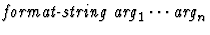). The first argument must be a string with exactly n occurrences of the characters ~A. The remaining arguments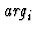are plugged in succession for each occurrence of ~A. • (%sym). The first argument must be a string with exactly n occurrences of the characters ~A. The remaining argumentsare plugged in succession for each occurrence of ~A and the resulting string is read in as a symbol. • (*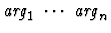). The set of assumptions (regarded as strings) of the current sequent node which match any argument. • (~*). The set of assumptions (regarded as strings) of the current sequent node which match no argument. • If the expression is a list whose first element is not an expression operator, its value is the list of values of the arguments. # 12.5 The Script Interpreter A script is a list (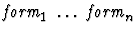of script expressions. The interpreter executes each form in the script in left-to-right order12.1. Once a form is executed by the script interpreter at a sequent node S, execution of the script continues at a new sequent node S1 called the natural continuation node of the script. This node is usually the first ungrounded relative of S after the form is executed. The only exception is when the form is a node motion keyword form. The rules for script interpreter execution are given below. Interpreting a script expression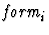usually causes side effects on the current deduction graph and other structures associated to the current proof. The side-effects depend on the kind of form being handled by the interpreter. ## Keyword Forms Recall that a keyword form is a script expression which is a list whose first element is a keyword. The execution of keyword forms depends on the keyword: • Node motion forms. These forms cause script execution to continue at a node other than the natural continuation node. The following are the node motion keyword forms: • (move-to-sibling n). This causes execution to proceed at the n-th sibling node. • (move-to-ancestor n). This causes execution to proceed at the n-th ancestor node. • (move-to-descendent (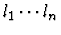)). This causes execution to proceed at the specified descendent node. Each li is an integer or a dotted pair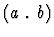of integers. • (jump-to-node label). label is a deduction graph sequent node label. • Conditionals. (if test-form consequent alternative) These are forms which provide conditional execution of command forms. The test-form can be of the following kinds: • (matches?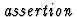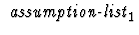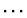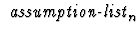), where assertion and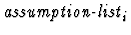are script expressions. The condition is true if the value of assertion matches the assertion of the current sequent node and every formula in the value of assumption-list matches an assumption of the current sequent node. • (minor?). True if the current sequent node is the minor premise of some inference node. • (major?). True if the current sequent node is the major premise of some inference node. • (generated-by-rule? rule-name). True if the current sequent node is an assumption of an inference node with name rule-name. • (succeeds? script-form). True if script-form applied to current sequent node grounds it. May cause side effects on the deduction graph (and is usually intended to do so.) • (progresses? script-form). True if script-form applied to current sequent node makes any progress. May cause side effects on the deduction graph (and is usually intended to do so.) • (not test-form). True if test-form fails. May cause side effects on the deduction graph. • (and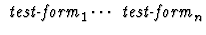). True if all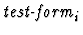are true. May cause side effects on the deduction graph. • (or). True if at least oneis true. May cause side effects on the deduction graph. • Blocks. (block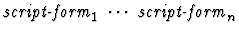). A block form merely provides a grouping for a list of script forms. • Iteration forms. These are of two kinds: • Range iterations provide for execution over a specified range of nodes. These have the form (for-nodes range-form script-form). range-form can be one of the following: • (unsupported-descendents). • (minor-premises). • (node-and-siblings). • Conditional iterations provide for execution while a specified condition holds. These have the form (while test-form script-form), where test-form is specified in the same way as for conditional forms. • Skip. (skip). This is a form used to insert in locations where at least one script form is required. It is essentially equivalent to (block). • Assignments. • (let-script name arg-count script). This form adds a command with name$name. The value of $name is this name in the current execution environment. • (let-macete name compound-macete-specification). This form adds a macete with name$name. The value of $name is this name in the current execution environment. • (let-val name expression). The value of$name is this name in the current execution environment.
• Deduction graph node labels.

• (label-node name). This form assigns the label name to the current node.
All these forms modify the current execution environment.
• Comments. (script-comment comment-string). Adds a comment to the preceding history entry. This keyword form has no other effect.

## Other Forms

Execution of any other form form in a script has the following result: The value of form must be a command form which is applied to the current node. In other words:
• If the value of form is a symbol, this value should be the name of a command which takes no arguments; otherwise an error results. The script interpreter then applies that command to the current sequent node.
• If the value of form is a list, the first expression of the list must be the name of a command, while the remaining elements should be arguments of the command; otherwise an error results. The script interpreter then applies that command with the resulting arguments to the current sequent node.

The following are possible arguments to a command:

• A symbol. Depending on the command and the location of the argument, this symbol may refer to a theorem, a macete, a translation, an inductor, a quasi-constructor, or a constant.

• A string. This string can be used to refer to an expression, a theorem, or a context.

• An integer. This integer can be used to refer to a sequent node, an occurrence number within an expression, or the number of an assumption.
• A list of strings.
• A list of integers.

# 12.6 Hints and Cautions

1.
To build a proof script for insertion in a def-theorem form, it is much easier to first prove the theorem interactively and then record the proof script using C-c i. A novice user should not attempt to build scripts from scratch without thoroughly understanding how scripts replay interactive proofs.
2.
More experienced users can build new scripts by modifying existing ones. For example, the following is a def-theorem form with a valid proof:
  (def-theorem MIN-LEMMA
"forall(a,b,c:rr,
a<b and a<c
implies
forsome(d:rr, a<d and d<=b and d<=c))"
(theory h-o-real-arithmetic)
(proof
(
direct-and-antecedent-inference-strategy
(instantiate-existential ("min(b,c)"))
(unfold-single-defined-constant-globally min)
(case-split ("b<=c"))
simplify
simplify
(apply-macete-with-minor-premises minimum-1st-arg)
(apply-macete-with-minor-premises minimum-2nd-arg)
)))

To get a valid proof for an analogous theorem where the arguments to < and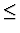are interchanged, one can edit the previous script replacing min by max everywhere in the proof:
   (def-theorem MAX-LEMMA
"forall(a,b,c:rr,
b<a and c<a
implies
forsome(d:rr, d<a and b<=d and c<=d))"
(theory h-o-real-arithmetic)
(proof
(
direct-and-antecedent-inference-strategy
(instantiate-existential ("max(b,c)"))
(unfold-single-defined-constant-globally max)
(case-split ("b<=c"))
simplify
simplify
(apply-macete-with-minor-premises maximum-1st-arg)
(apply-macete-with-minor-premises maximum-2nd-arg)
)))Next: 13. Simplification Up: 2. User's Guide Previous: 11. Theory Ensembles